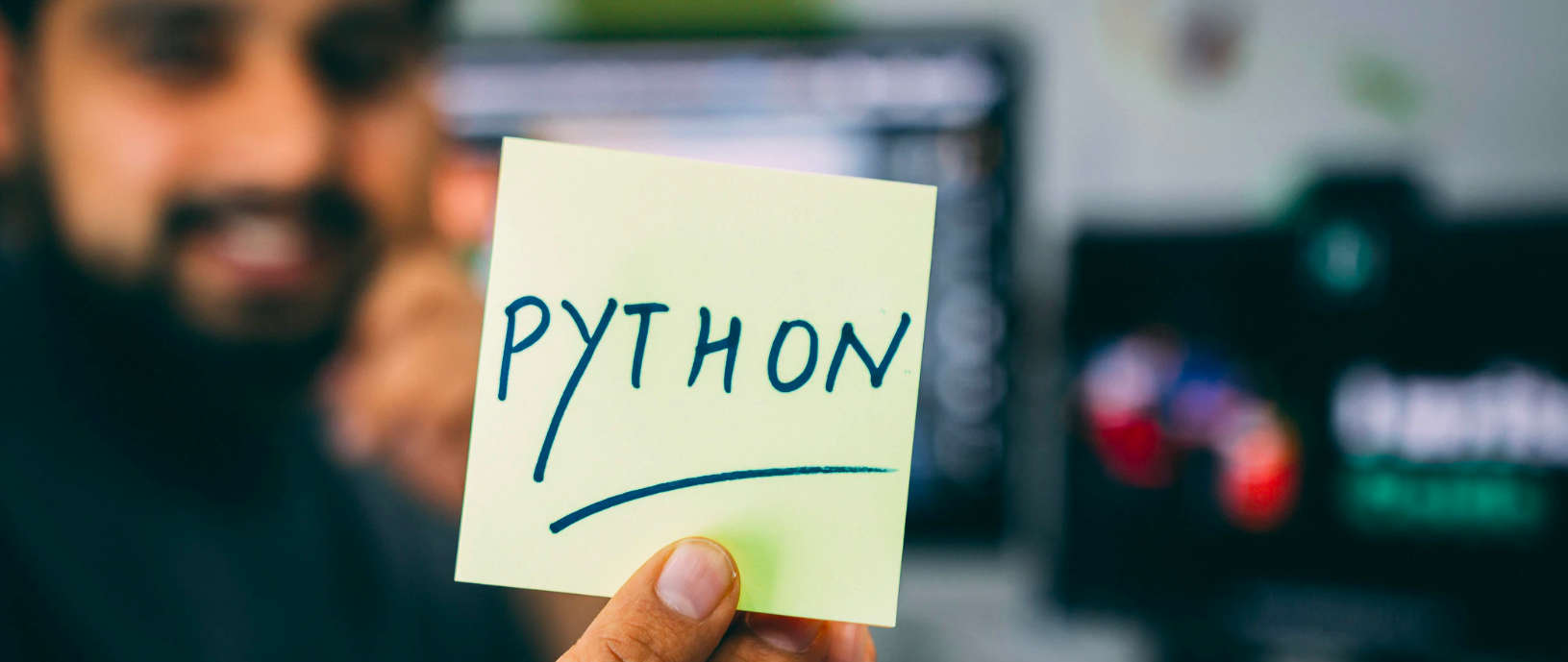Santi

• 5

获得赞
• 2

发布的文章
• 0

答辩的项目

## Python子进程subprocess调用二三事

Python3

Python3

1. Python是目前机器学习、深度学习与AI竞赛的主流语言。
2. 在使用Python能加比赛时，时常需要调用子进程进行并行数据处理，subprocess其中的一些坑不容易爬起来，这里为大家填坑，欢迎留言交流。```import subprocess

cmd = ['ls']
process = subprocess.Popen(cmd, stdout=subprocess.PIPE)
print(stdout_line)
process.stdout.close()
return_code = process.wait()
if return_code:
raise subprocess.CalledProcessError(return_code, cmd)```

shell=True

```import subprocess

cmd = ['dir']
process = subprocess.Popen(cmd, stdout=subprocess.PIPE, shell=True)
print(stdout_line)```

```my_env = os.environ.copy()
my_env['LD_LIBRARY_PATH'] = '../libs/x64/'

cmd = ['command']
popen = subprocess.Popen(cmd,
stdout=subprocess.PIPE,
env=my_env)```

```pwd = os.path.dirname(__file__)

cmd = ['command']
popen = subprocess.Popen(cmd,
stdout=subprocess.PIPE,
cwd=os.path.join(pwd, '..', 'bin'))```

500字Santi

• 5

获得赞
• 2

发布的文章
• 0

答辩的项目